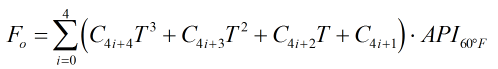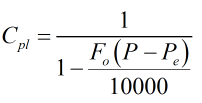# C298 – Product Type 7 – Cpl and Compressibility Calculation

## Description

This calculates the Cpl and compressibility factor for a crude oil according to the Aramco equations for product type 7. It uses the density/specific gravity/API gravity at 60°F of the sample, the temperature and pressure as well as equilibrium pressure of the liquid.

FMC Energy Systems – GeoFlo II – DMR – Flow computer manual. – Bulletin MN09034L

FMC Energy Systems – Smith Meter GeoProv – Bidirectional Prover Computer Manual. – Bulletin MN09019L

Aramco Product Type 7 equations

Kelton calculation reference C298

FLOCALC calculation reference F096

## Options

### Standard Density Input

• Density
• Specific gravity
• API gravity

This option is used to select whether standard density at 60°F will be entered as a density, specific gravity or API gravity.

## Constants

 Coefficients for Product Type 7 C1 = 2.10222 x 10-2 C2 = 6.09156 x 10-5 C3 = 5.18064 x 10-8 C4 = 4.25526 x 10-9 C5 = 4.42976 x 10-4 C6 = 4.30509 x 10-6 C7 = -2.69122 x 10-8 C8 = -8.77856 x 10-11 C9 = -5.18890 x 10-6 C10 = -5.37873 x 10-8 C11 = -6.43473 x 10-11 C12 = 1.94889 x 10-11 C13 = 1.80682 x 10-7 C14 = 1.69756 x 10-9 C15 = -1.83219 x 10-11 C16 = -2.21778 x 10-13 C17 = -7.57157 x 10-10 C18 = -7.26725 x 10-12 C19 = 1.56277 x 10-13 C20 = 9.04520 x 10-16

## Calculation

### Compressibility Factor

The compressibility factor is calculated by:Where API60 = API gravity at 60°F T = Temperature C = Coefficients for compressibility equation

### Correction Factor for Pressure

The correction factor for pressure on the liquid, Cpl, is calculated by:Where P = Pressure Pe = Equilibrium pressure

Back